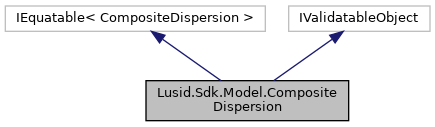LUSID C# SDK
Lusid.Sdk.Model.CompositeDispersion Class Reference

A list of Dispersion calculations for the given years. More...

Inheritance diagram for Lusid.Sdk.Model.CompositeDispersion:[legend]

## Public Member Functions

CompositeDispersion (DateTimeOffset effectiveAt=default(DateTimeOffset), decimal dispersionCalculation=default(decimal), decimal variance=default(decimal), decimal firstQuartile=default(decimal), decimal thirdQuartile=default(decimal), decimal range=default(decimal), List< ResourceId > constituentsInScope=default(List< ResourceId >), List< ResourceId > constituentsExcluded=default(List< ResourceId >))
Initializes a new instance of the CompositeDispersion class. More...

override string ToString ()
Returns the string presentation of the object More...

virtual string ToJson ()
Returns the JSON string presentation of the object More...

override bool Equals (object input)
Returns true if objects are equal More...

bool Equals (CompositeDispersion input)
Returns true if CompositeDispersion instances are equal More...

override int GetHashCode ()
Gets the hash code More...

## Protected Member Functions

CompositeDispersion ()
Initializes a new instance of the CompositeDispersion class. More...

## Properties

DateTimeOffset EffectiveAt` [get, set]`
The date for which dipsersion calculation has been done. This should be 31 Dec for each given year. More...

decimal DispersionCalculation` [get, set]`
The result for the dispersion calculation on the given effectiveAt. More...

decimal Variance` [get, set]`
The variance on the given effectiveAt. More...

decimal FirstQuartile` [get, set]`
First Quartile (Q1) &#x3D; (lower quartile) &#x3D; the middle of the bottom half of the returns. More...

decimal ThirdQuartile` [get, set]`
Third Quartile (Q3) &#x3D; (higher quartile) &#x3D; the middle of the top half of the returns. More...

decimal Range` [get, set]`
Highest return - Lowest return. More...

List< ResourceIdConstituentsInScope` [get, set]`
List containing Composite members which are part of the dispersion calcualtion. More...

List< ResourceIdConstituentsExcluded` [get, set]`
List containing the Composite members which are not part of the dispersion calculation More...

## Detailed Description

A list of Dispersion calculations for the given years.

## ◆ CompositeDispersion() [1/2]

 Lusid.Sdk.Model.CompositeDispersion.CompositeDispersion ( )
inlineprotected

Initializes a new instance of the CompositeDispersion class.

## ◆ CompositeDispersion() [2/2]

 Lusid.Sdk.Model.CompositeDispersion.CompositeDispersion ( DateTimeOffset effectiveAt = `default(DateTimeOffset)`, decimal dispersionCalculation = `default(decimal)`, decimal variance = `default(decimal)`, decimal firstQuartile = `default(decimal)`, decimal thirdQuartile = `default(decimal)`, decimal range = `default(decimal)`, List< ResourceId > constituentsInScope = `default(List)`, List< ResourceId > constituentsExcluded = `default(List)` )
inline

Initializes a new instance of the CompositeDispersion class.

Parameters
 effectiveAt The date for which dipsersion calculation has been done. This should be 31 Dec for each given year. (required). dispersionCalculation The result for the dispersion calculation on the given effectiveAt. (required). variance The variance on the given effectiveAt. (required). firstQuartile First Quartile (Q1) = (lower quartile) = the middle of the bottom half of the returns. (required). thirdQuartile Third Quartile (Q3) = (higher quartile) = the middle of the top half of the returns. (required). range Highest return - Lowest return. (required). constituentsInScope List containing Composite members which are part of the dispersion calcualtion.. constituentsExcluded List containing the Composite members which are not part of the dispersion calculation.

## ◆ Equals() [1/2]

 bool Lusid.Sdk.Model.CompositeDispersion.Equals ( CompositeDispersion input )
inline

Returns true if CompositeDispersion instances are equal

Parameters
 input Instance of CompositeDispersion to be compared
Returns
Boolean

## ◆ Equals() [2/2]

 override bool Lusid.Sdk.Model.CompositeDispersion.Equals ( object input )
inline

Returns true if objects are equal

Parameters
 input Object to be compared
Returns
Boolean

## ◆ GetHashCode()

 override int Lusid.Sdk.Model.CompositeDispersion.GetHashCode ( )
inline

Gets the hash code

Returns
Hash code

## ◆ ToJson()

 virtual string Lusid.Sdk.Model.CompositeDispersion.ToJson ( )
inlinevirtual

Returns the JSON string presentation of the object

Returns
JSON string presentation of the object

## ◆ ToString()

 override string Lusid.Sdk.Model.CompositeDispersion.ToString ( )
inline

Returns the string presentation of the object

Returns
String presentation of the object

## ◆ ConstituentsExcluded

 List Lusid.Sdk.Model.CompositeDispersion.ConstituentsExcluded
getset

List containing the Composite members which are not part of the dispersion calculation

List containing the Composite members which are not part of the dispersion calculation

## ◆ ConstituentsInScope

 List Lusid.Sdk.Model.CompositeDispersion.ConstituentsInScope
getset

List containing Composite members which are part of the dispersion calcualtion.

List containing Composite members which are part of the dispersion calcualtion.

## ◆ DispersionCalculation

 decimal Lusid.Sdk.Model.CompositeDispersion.DispersionCalculation
getset

The result for the dispersion calculation on the given effectiveAt.

The result for the dispersion calculation on the given effectiveAt.

## ◆ EffectiveAt

 DateTimeOffset Lusid.Sdk.Model.CompositeDispersion.EffectiveAt
getset

The date for which dipsersion calculation has been done. This should be 31 Dec for each given year.

The date for which dipsersion calculation has been done. This should be 31 Dec for each given year.

## ◆ FirstQuartile

 decimal Lusid.Sdk.Model.CompositeDispersion.FirstQuartile
getset

First Quartile (Q1) &#x3D; (lower quartile) &#x3D; the middle of the bottom half of the returns.

First Quartile (Q1) &#x3D; (lower quartile) &#x3D; the middle of the bottom half of the returns.

## ◆ Range

 decimal Lusid.Sdk.Model.CompositeDispersion.Range
getset

Highest return - Lowest return.

Highest return - Lowest return.

## ◆ ThirdQuartile

 decimal Lusid.Sdk.Model.CompositeDispersion.ThirdQuartile
getset

Third Quartile (Q3) &#x3D; (higher quartile) &#x3D; the middle of the top half of the returns.

Third Quartile (Q3) &#x3D; (higher quartile) &#x3D; the middle of the top half of the returns.

## ◆ Variance

 decimal Lusid.Sdk.Model.CompositeDispersion.Variance
getset

The variance on the given effectiveAt.

The variance on the given effectiveAt.

The documentation for this class was generated from the following file: